# Atoms and Molecules Notes Class 9th Science Chapter 3

0
2692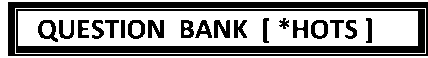CHAPTER – 3 Atoms and Molecules _concept details

KEY CONCEPTS : [ *rating as per the significance of concept]

 1. Laws of Chemical Combination *** 2. John Daltons Atomic Theory ** 3. Atoms, ions & Chemical Formula **** 4. Mole Concept ***** 5. Molar Mass & Avogadro constant ****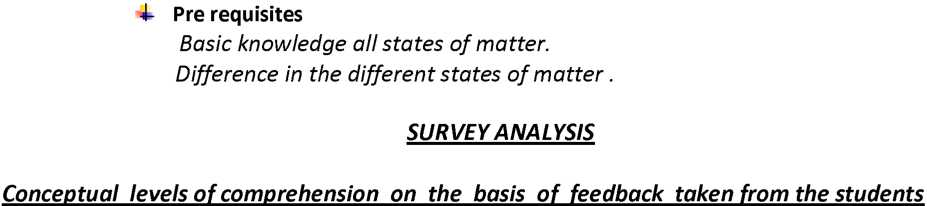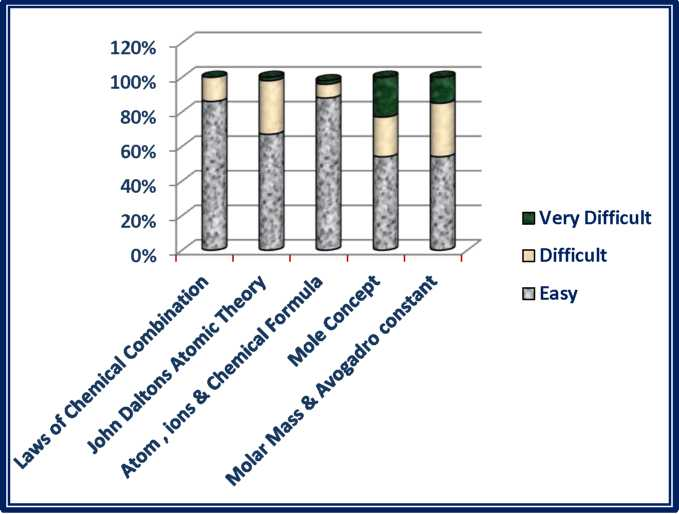Verification of “Law of Conservation of mass”

l.Laws of Chemical Combination |

A solution of sodium chloride and silver nitrate are taken separately in the two limbs of an ‘H’ shaped tube. The tube is sealed and weighed precisely. The two reactants are made to react by inverting the tube. The following reaction takes place.

AgNO3(aq) + NaCl (aq) -> AgCl (s) + NaNO3 (aq)

The whole tube is kept undisturbed for sometime so that the reaction is complete. When the tube is weighed again it is observed that:

Weight before the reaction = Weight after the reaction Limitation of “Law of definite proportion”

This law does not hold good when the compound is obtained by using different isotopes of the combining elements .

Q.1 Why chemical reactions are in accordance with the Law of conservation of mass? Q.2 Calculate the ratio of atoms present in 5 g of magnesium and 5 g of iron.

[Atomic mass of Mg=24 u, Fe=56 u]

1. John Daltons Atomic Theory |

[ for postulates ,refer NCERT text book article 3.1.2 -page no.32 ]

Using his theory, Dalton rationalized the various laws of chemical combination which were in existence at that time. However, he assumed that the simplest compound of two elements must be binary.

Q.1 In what respect does Dalton’s Atomic theory hold good even today?

Q.2 Which of the following is not the postulate of Dalton’s Atomic theory of matter ?

1. Each element is made up of extremely small particles called atoms.
2. Atoms of a given element are identical in chemical properties but have different

physical properties.

1. Atoms cannot be created nor destroyed.
2. Compounds are formed by the chemical union of atoms of two or more elements in fixed proportion .
3. Atoms ,Molecules, Ions & Chemical Formula |
 Atom Molecule -o- An atom is the smallest particle of an element which can take part in a chemical reaction. It may or may not exist freely. The smallest particle of matter (element or compound) which can exist in a free state. -0- O Each atom of an element shows all the properties of the element. The properties of a substance are the properties of its molecules.
• MOLECULES OF ELEMENT: The molecules of an element are constituted by the same type of atoms.
• MOLECULES OF COMPOUND: Atoms of different elements join together in definite proportions to form molecules of compounds.(hetero atomic molecules)
• ATOMICITY : The number of atoms contained in a molecule of a substance (element or compound) is called its atomicity.
 Element Formula Atomicity Ozone O3 3 Phosphorus P4 4 Sulphur S8 8 Oxygen O2 2
• Based upon atomicity molecules can be classified as follows.

Monoatomic molecules: Noble gases helium, neon and argon exist as He Ne and Ar

respectively.

Diatomic molecules: H2 , O2, N2,Cl2, CO , HCl .

Triatomic molecules: O3 ,CO2 , NO2.

• SYMBOLS
• The abbreviation used to represent an element is generally the first letter in capital of the English name of element.

Oxygen -> O Nitrogen -> N

• When the names of two or more elements begin with the same initial letter, the initial letter followed by the letter appearing later in the name is used to symbolize the element

Barium -> Ba Bismuth -> Bi

Symbols of some elements are derived from their Latin names

 ELEMENT LATIN NAME SYMBOL Sodium Natrium Na Copper Cuprum Cu Potassium Kalium K Iron Ferrum Fe Mercury Hydragyrum Hg Tungsten Wolfram W

Q.1 Give one example each of molecule of element & molecule of compound.

Q.2 How does an atom differ from molecule ?

Q.3 Name a triatomic gas.

Q.4 Name the element represented by Hg, Pb, Au.

Q.5 What is the difference between an atom of hydrogen and a molecule of hydrogen?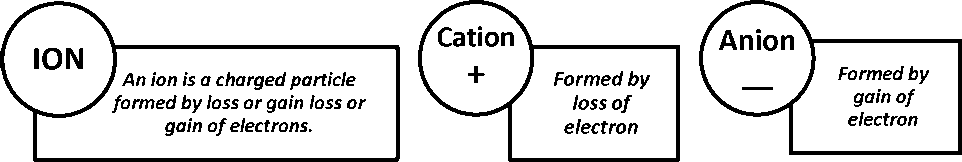Polyatomic Ion : A group of atoms carrying a charge is as polyatomic ion.

eg: NH4+ – Ammonium Ion ; CO32- – Carbonate ion

Valency : The number of electrons which an atom can lose , gain or share to form a bond.

OR

It is the combining capacity of an atom of the element.

[ for valency of various cations & anions ,refer NCERT text book table 3.6, page no. 37 ]

♦♦♦ Chemical Formula: A chemical formula is a short method of representing chemical elements and compounds.

Writing a Chemical Formula -CRISS-CROSS rule

[ b]

 RULE 1 [a] write the correct symbols of two elements. Ex : Aluminium & Oxygen Al O

above each symbol, write the correct valence

Al3+ O2-

[c]

Criss-cross the valence and drop the algebraic sign.

Al2O3

 RULE 2 > When the subscript is number 1, subscript is not written. Ex. Sodium Chloride – Na1+ Cl1- — NaCl

RULE 3> When the valence of both elements are numerically equal , the subscripts are also not written.

Ex. Calcium Oxide- – Ca2+ O2- — CaO

RULE 4 > When there are multiple numbers of an individual polyatomic ion ,

parentheses must be used to separate the polyatomic ion from the subscirpt.

Ex. Ammonium Sulphate- – NH41+ SO/ ‘ (NH4)2 SO4

EXAMPLES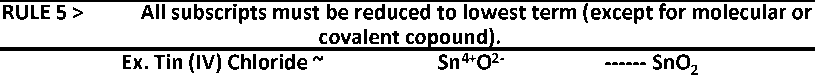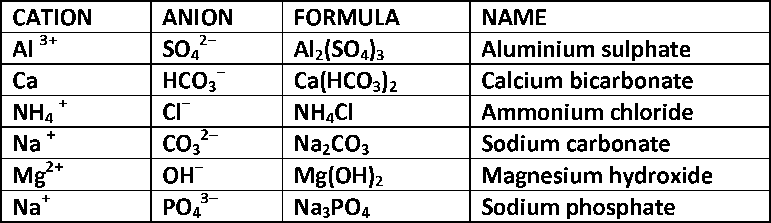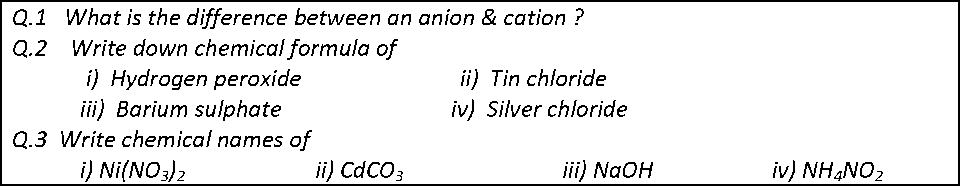1. Mole Concept \

The mole (mol) is the amount of a substance that contains as many elementary entities as there are atoms in exactly 12.00 grams of 12C

The Avogadro constant is named after the early nineteenth century Italian scientist Amedeo Avogadro.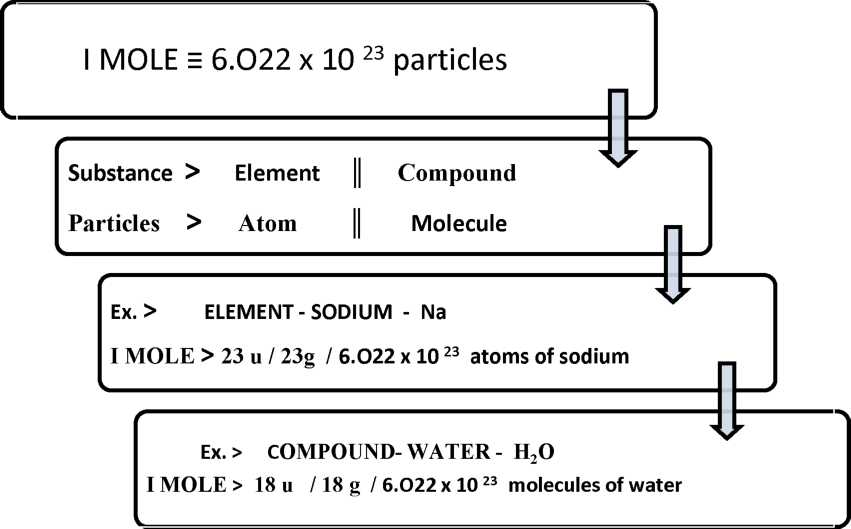S.No. Symbol / formula of atom / molecule Atomic / molecular mass ( u) lmole ( in g) Avogadro no. Molar mass ( g mol -1) 1. O 16 u 16 g 6.022 x 1023 atoms 16 g mol -1 2. Nz 28 u 28 g 6.022 x 1023 molecules 28 g mol -1 3. HCl 36.5 u 36.5 g 6.022 x 1023 molecules 36.5 g mol -1

GRAM MOLECULAR MASS

Gram molecular mass is the mass in grams of one mole of a molecular substance.

Ex: The molecular mass of N2 is 28, so the gram molecular mass of N2 is 28 g.

ATOMIC MASS UNIT

An atomic mass unit or amu is one twelfth of the mass of an unbound atom of carbon-12. It is a unit of mass used to express atomic masses and molecular masses.

Also Known As: Unified Atomic Mass Unit (u).

MOLECULAR MASS : A number equal to the sum of the atomic masses of the atoms in a molecule. The molecular mass gives the mass of a molecule relative to that of the 12C atom, which is taken to have a mass of 12.

Examples: The molecular mass of C2H6 is approximately 30 or [(2 x 12) + (6 x 1)]. Therefore the molecule is about 2.5 times as heavy as the 12C atom or about the same mass as the NO atom with a molecular mass of 30 or (14+16).

Q.1 What term is used to represent the mass of 1 mole molecules of a substance? Q.2 What is the gram atomic mass of i) Hydrogen ii) oxygen ?

Q.3 Calculate molar mass of C2H2.

1. Molar Mass & Avogadro Constant |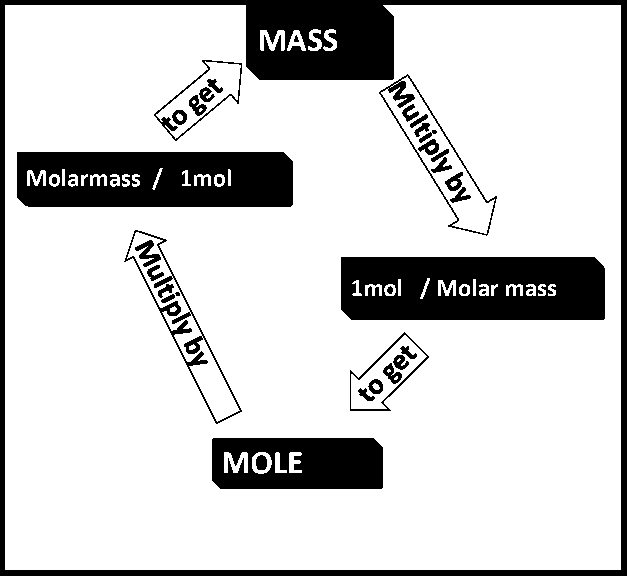Ex: i) Convert 35 g of Al into mol.

A: Molar mass of Al= 27 g

27 g = 1mol

1mol

= 35 g x

27 g

= 1.3 mol of Al

ii) How many grams of SiO2 are present in 0.8 mol ?

A: Molar mass of SiO2 = 60.1 g

1 mol = 60.1 g

60.1g of SiO2

= 0.8 mol of SiO2 x

1mol of SiO2

= 48.1 g SiO2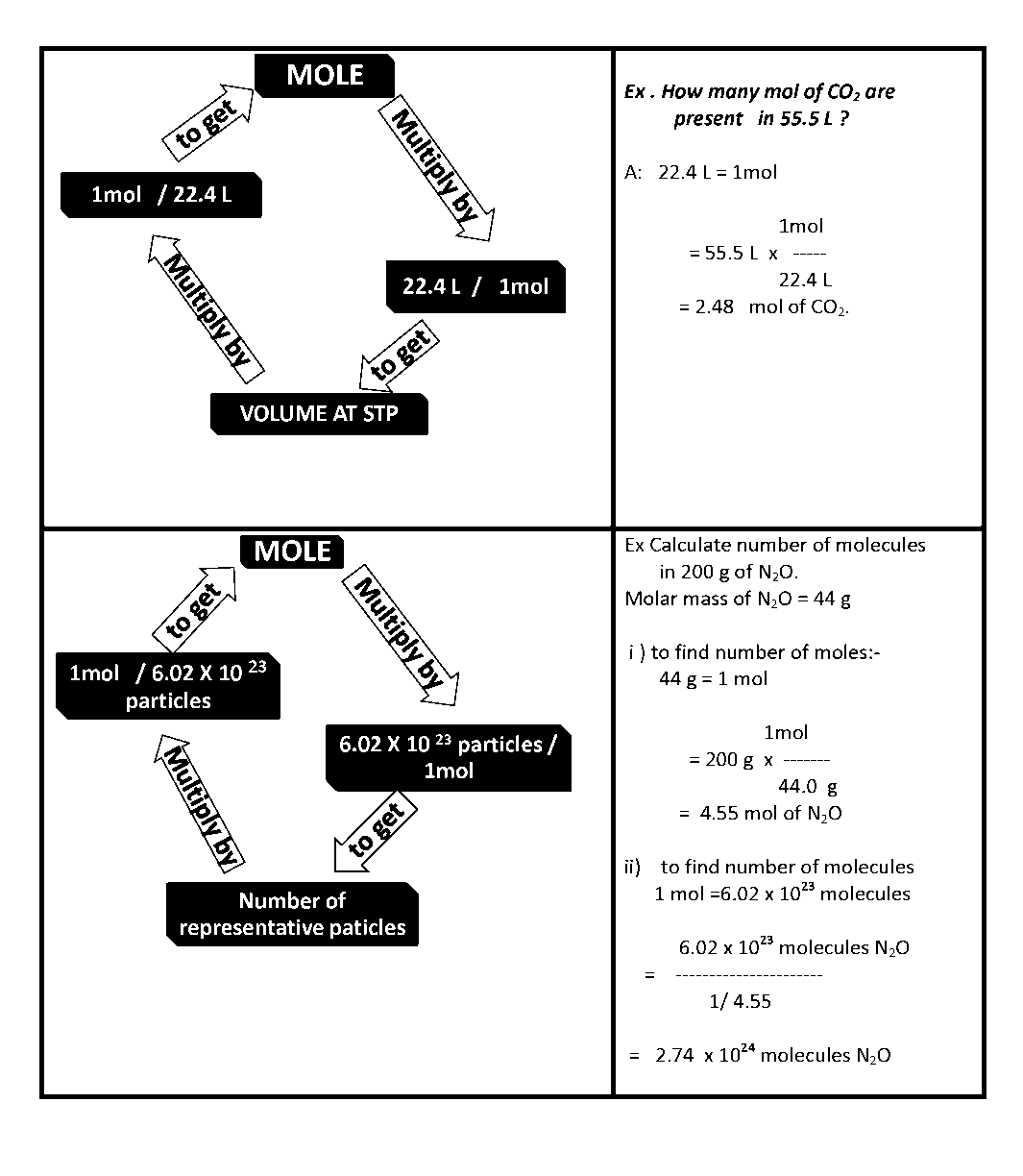Q.1 Calculate the mass of one atom of sodium?

Q.2 The atomic mass of calcium is 40 u. What will be the number of calcium atoms in 0.4 u of calcium?

Q.3 How many atoms of oxygen are present in 120 g of nitric acid ?1. Mark Questions:
2. Who gave law of conservation of mass ?
3. What term is used to represent the mass of 1 mole molecules of a substance?
4. What name is given to the number 6.023 x 10 23 ?
5. What is molecular mass?
6. Give Latin names for sodium & mercury.
7. *How many atoms are there in exactly 12 g of carbon ?
8. Define mole.
9. Calculate formula unit mass of CaCl2. [ At. Mass : Ca = 40 u , Cl = 35.5 u ]
10. Name a diatomic gas.
11. How many atoms are present in H2SO4.
12. Marks Questions:
13. Give the chemical symbols for the following elements: Gold, Copper , Potassium & Iron.
14. *What do the following symbols represent – i) 1 H & i) H2
15. Neon gas consists if single atoms , what mass of neon contain 6.022 x 1023 atoms.
16. What elements do the following compounds contain ? i) Water ii) Lead nitrate
17. State the differences between an atom or a molecule.
18. Molar Mass of water is 18 g mol-1 , what is the mass of 1 mole of water? .
19. *The number of atoms in 1 mole of hydrogen is twice the number of atoms in one mole of helium. Why?
20. Write the chemical formulas for the following:

i) Silver oxide ii) Iron (III) sulphate

1. Calculate molar mass of H2O2 & HNO3.
2. What is the mass of 0.2 moles of oxygen molecules?
3. Marks Questions:
4. State the main postulates of John Dalton’s atomic theory.
5. What are polyatomic ions ? Give two examples.
6. State the following
7. Law of conservation of mass. ii) Law of constant proportion
8. What is the mass of :
9. 1 mol of N atoms. ii) 4 mol of Al atoms.
10. What is meant by the term atomicity ? State the atomicity of i) Phosphorous
11. Sulphur

5 Marks Questions:

1. i) What is molecular formula ? State with example what information can be derived from a molecular formula .
2. Write the names of the compounds represented by the following formulas: a) Mg(NO3)2 b) K2SO4 c )Ca3N2
3. * i) What is gram molecular mass?
4. Write the formulas & names of the compounds formed between :

a) Ferrous and sulphide ions b) Aluminium and sulphate ions

1. Potassium and chlorate ions d) Barium and chloride ions
2. i) Calculate the number of moles for the following:

a) 52 g of He b) 17 g of H2O

1. How many molecules are present in 34 g of ammonia ?
2. Calculate the mass of 0.5 mole of sugar (C12H22O11).

You Are expected to know..

• Laws of Chemical combination.
• John Dalton’s imagination about atom & the limitation of his theory.
• Difference between an atom & molecule.
• Types of ions
• Writing chemical formula of compounds.
• Relationship between Mole , Molar Mass & Avogadro Constant